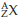×
Get Full Access to Introductory Chemistry - 5 Edition - Chapter 4 - Problem 100p
Get Full Access to Introductory Chemistry - 5 Edition - Chapter 4 - Problem 100p

×

# Solved: Plutonium-239 is used in nuclear bombs. Determine the number of protons andISBN: 9780321910295 34

## Solution for problem 100P Chapter 4

Introductory Chemistry | 5th Edition

• Textbook Solutions
• 2901 Step-by-step solutions solved by professors and subject experts
• Get 24/7 help from StudySoup virtual teaching assistantsIntroductory Chemistry | 5th Edition

4 5 1 333 Reviews
28
4
Problem 100P

Plutonium-239 is used in nuclear bombs. Determine the number of protons and neutrons in plutonium-239 and write its symbol in the form.

Step-by-Step Solution:
Step 1 of 3

Solution 100P

Here, we are going to calculate the number of protons and neutrons in plutonium-239

When atoms have same atomic number but different mass number are called isotopes. Isotopes can be symbolized by using the following notation.Where, X is the chemical symbol

A is the mass number = Number of protons + number of neutrons

Z is the atomic number = Number of protons present in the nucleus of that atom.

Since, plutonium-239 is one of the isotope of plutonium.

The  atomic number of plutonium  Z = 94

Thus, number of protons of plutonium atom = 94

The mass number of carbon plutonium-239 = 239

Therefore,

Mass...

Step 2 of 3

Step 3 of 3

##### ISBN: 9780321910295

The full step-by-step solution to problem: 100P from chapter: 4 was answered by , our top Chemistry solution expert on 05/06/17, 06:45PM. This full solution covers the following key subjects: plutonium, nuclear, form, its, Neutrons. This expansive textbook survival guide covers 19 chapters, and 2045 solutions. Since the solution to 100P from 4 chapter was answered, more than 296 students have viewed the full step-by-step answer. This textbook survival guide was created for the textbook: Introductory Chemistry, edition: 5. Introductory Chemistry was written by and is associated to the ISBN: 9780321910295. The answer to “Plutonium-239 is used in nuclear bombs. Determine the number of protons and neutrons in plutonium-239 and write its symbol in the form .” is broken down into a number of easy to follow steps, and 23 words.

Unlock Textbook Solution